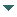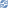# gvSIG

##### Personal tools
You are here: Home Geometry examples
gvSIG DesktopgvSIG Desktop

Cached time 11/21/13 17:27:08# Geometry examples

Up one level
 ◄ Previous Geometry creation Next: Point ►

Examples about how to create a determinated-type geometries and how to edit their coordinate values.

### Point

To create a geometry of the Point type, is necessary to use the code Point of the list on TYPES. We can use the generic method from the GeometryManager to create the geonometry, to do a casting to Point and to assign the values. For example, to create a 2-dimensional geometry with the coordinates 2,4 we need to execute the following code:

```Point point = (Point) geometryManager.create(TYPES.POINT, SUBTYPES.GEOM2D);
point.setX(2);
point.setY(4);
```

There're directly methods both to write (setX and setY) and to read (getX and getY) the first and the second coordenate. To edit or recuperate the rest of values from the other dimensions you can use the generic methods setCoordinateAt and getCoordinateAt.

The manager has directly methods to create the most common geometries with the intent that we don't need to do a casting and the assignation of the values to the two first coordinates. In the Point case, we can use the method createPoint from the manager, where we must indicate the subtype of the point and the values of the first and second coordenate.

```Point point = geometryManager.createPoint(2, 4, SUBTYPES.GEOM2D);
```

There aren't specific methods for all the kinds of geometries. For example, to create a 3-dimensional point which has the coordenates 1,1,5 we must execute the following code:

```Point point = geometryManager.createPoint(1, 1, SUBTYPES.GEOM3D);
point.setCoordinateAt(2, 5);
```

Another option could be do it for each coordenate, and the result point will be the same:

```Point point = (Point) geometryManager.create(TYPES.POINT, SUBTYPES.GEOM2D);
point.setX(1);
point.setY(1);
point.setCoordinateAt(2, 5);
```

### Curve

To create a curve we can use the generic method create from the manager. To establish the coordenate's values we can use an object of the GeneralPathX type that is also available because the backward compatibility. The steps to create a polyline from the point 5,5 to the 10,10 are this:

```Curve curve = (Curve)geometryManager.create(TYPES.CURVE, SUBTYPES.GEOM2D);
GeneralPathX generalPathX = new GeneralPathX();
generalPathX.moveTo(5, 5);
generalPathX.lineTo(10, 10);
curve.setGeneralPath(generalPathX);
```

A curve must be created from the own model's objects. In the curve case, they will be Point type objects. The following code let us to create a curve with the same coordentes like the previous example:

```Point point1 = geometryManager.createPoint(5, 5, SUBTYPES.GEOM2D);
Point point2 = geometryManager.createPoint(10, 10, SUBTYPES.GEOM2D);
Curve curve = (Curve)geometryManager.create(TYPES.CURVE, SUBTYPES.GEOM2D);
```

The addVertex method inserts a new vertex in the last position in a Curve object and it should be used in the geometry creation. In the other hand, there're more methods which let us the edition of the curve's coordenates. For example, if we want to change the final point of the curve in the last example, we could do this:

```Point endPoint = geometryManager.createPoint(15, 15, SUBTYPES.GEOM2D);
curve.setVertex(1, endPoint);
```

The setVertex method fixes the value of one of the internal points which are part of the curve composition and replace it for the point which was previously. In the other hand, we can also insert a point inside the curve. For example, to insert the point 7,7 in the second position we must execute the following code:

```Point middlePoint = geometryManager.createPoint(7, 7, SUBTYPES.GEOM2D);
curve.insertVertex(1, middlePoint);
```

The first parameter is the point position that we want to put in. In this case, the resultant curve will have the coordinates 5,5 7,7 and 15,15 such as we have been defining.

### Arc

An arc can be created from a central point which works like a radius and another two that will be used to determine the beginning and the end of the arc. In the next example, we will create an arc with the point 0,0 for the radius and from the point 1,0 to the 0,1:

```Point centerPoint = geometryManager.createPoint(0, 0, SUBTYPES.GEOM2D);
Point startPoint = geometryManager.createPoint(1, 0, SUBTYPES.GEOM2D);
Point endPoint = geometryManager.createPoint(0, 1, SUBTYPES.GEOM2D);
Arc arc = (Arc)geometryManager.create(TYPES.ARC, SUBTYPES.GEOM2D);
arc.setPoints(centerPoint, startPoint, endPoint);
```

Moreover this way, an arc can be created from a initial point, a radius length and two angles. The next example creates an arc like the created in the previous example, with radius 1 and the beginning on 1,0 (0º) and finnish on 0,1 (90º):

```Point centerPoint = geometryManager.createPoint(0, 0, SUBTYPES.GEOM2D);
Arc arc = (Arc)geometryManager.create(TYPES.ARC, SUBTYPES.GEOM2D);
arc.setPoints(centerPoint, 1, 0, 90);
```

### Surface

To create a surface we can use the generic method create from the manager. To establish the coordinate's values, we can use a GeneralPathX type object. The steps to create a triangular polygon on the coordinates 0,0 0,5 5,5 can be the next:

```Surface surface = (Surface)geometryManager.create(TYPES.SURFACE, SUBTYPES.GEOM2D);
GeneralPathX generalPathX = new GeneralPathX();
generalPathX.moveTo(0, 0);
generalPathX.lineTo(0, 5);
generalPathX.lineTo(5, 5);
surface.setGeneralPath(generalPathX);
```

Like it happens on the Curve, a Surface must be breated from the own model's objects. In the polygon case, they will be Point objects. The next code let us to create a polygon with the same coordinates like the previous example:

```Point point1 = geometryManager.createPoint(0, 0, SUBTYPES.GEOM2D);
Point point2 = geometryManager.createPoint(0, 5, SUBTYPES.GEOM2D);
Point point3 = geometryManager.createPoint(5, 5, SUBTYPES.GEOM2D);
Surface surface = (Surface)geometryManager.create(TYPES.SURFACE, SUBTYPES.GEOM2D);
```

The addVertex method inserts a new vertex in the last position in a Surface object and it should be used in the geometry creation. In the other hand, there're more methods which let us the edition of the internal vertex's coordenates that compose the polygon. For example, if we want to change the final point of the polygon in the last example, we could do this:

```Point endPoint = geometryManager.createPoint(0, 10, SUBTYPES.GEOM2D);
surface.setVertex(2, endPoint);
```

The setVertex method fixes the value of one of the internal points which are part of the polygon composition and replace it for the point which was previously. The first parameter is the internal point position that is necessary to modify.

In the other hand, we can also insert a point inside the polygon. For example, to insert the point 0,7 in the third position we must execute the following code:

```Point middlePoint = geometryManager.createPoint(0, 7, SUBTYPES.GEOM2D);
surface.insertVertex(2, middlePoint);
```

The first parameter is the point position that we want to put in. In this case, the resultant polygon will have the coordinates 0,0 0,5 0,7 and 0,10 such as we have been defining.

### Circle

The circles are represented on gvSIG with the Circle interface. To create a circle we can use the generic method create from the manager and, after that, establish the values of the center and radius. If we have a Point object (0,0 in this example) and we know the radius (5), we can create a circle with the following steps:

```Point centerPoint = geometryManager.createPoint(0, 0, SUBTYPES.GEOM2D);
Circle circle = (Circle)geometryManager.create(TYPES.CIRCLE, SUBTYPES.GEOM2D);
circle.setPoints(centerPoint, 5);
```

Another option is to create a circle with a center and a point in the circle's circumference diameter. The next example let us to create a circle like the created in the first example:

```Point centerPoint = geometryManager.createPoint(0, 0, SUBTYPES.GEOM2D);
Point radiousPoint = geometryManager.createPoint(0, 5, SUBTYPES.GEOM2D);
Circle circle = (Circle)geometryManager.create(TYPES.CIRCLE, SUBTYPES.GEOM2D);
```

The third option is to create a circumference with three points because there's only one which pass on them. To do that, we must create the three points and to invoke the setPoints method:

```Point point1 = geometryManager.createPoint(0, 0, SUBTYPES.GEOM2D);
Point point2 = geometryManager.createPoint(5, 5, SUBTYPES.GEOM2D);
Point point3 = geometryManager.createPoint(7, 7, SUBTYPES.GEOM2D);
Circle circle = (Circle)geometryManager.create(TYPES.CIRCLE, SUBTYPES.GEOM2D);
circle.setPoints(point1, point2, point3);
```